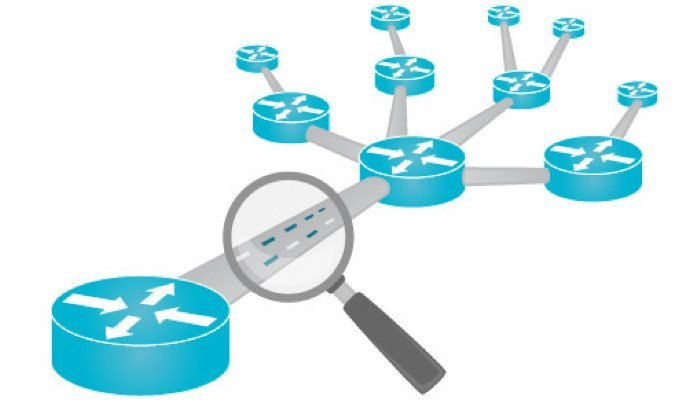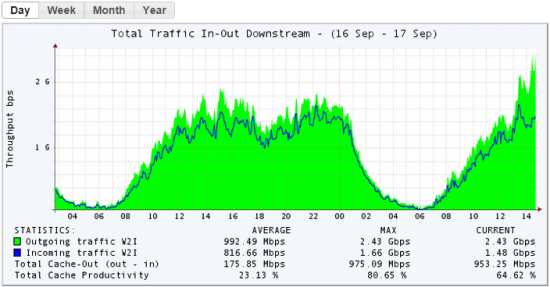# 数据业务流量的计量单位及换算

[来源：纤亿通]  [日期：2019-01-25]  [点击数：3316]bit与Byte之间可以进行换算，其换算关系为：
1Byte=8bit（或简写为：1B=8b，中文读法：1字节= 8比特），它们的进率为8；b是bit的缩写，即“位”，是信息技术中的最小存储单位，一位代表一个“1”或者“0”；B是Byte的缩写，即“字节”，是信息技术中一种较小的存储单位，一个英文字符占一个字节，一个汉字则占两个字节。1B = 8b

1 MB = 8 Mb

1Mbps = 0.125 M Byte/s = 1/8M Byte/s * 1024 = 128 KB/s

Tips:1KB (Kilobyte 千字节)=1024B,

1MB (Megabyte 兆字节 简称“兆”)=1024KB，

1GB (Gigabyte 吉字节 又称“千兆”)=1024MB,

1TB (Terabyte 万亿字节 太字节)=1024GB,

1PB (Petabyte 千万亿字节 拍字节)=1024TB,

1EB (Exabyte 百亿亿字节 艾字节)=1024PB,

1ZB (Zettabyte 十万亿亿字节 泽字节)= 1024 EB,

1YB (Yottabyte 一亿亿亿字节 尧字节)= 1024 ZB,

1BB (Brontobyte 一千亿亿亿字节)= 1024 YB.

yotta, [尧]它, Y. 10^24,

zetta, [泽]它, Z. 10^21,

exa, [艾]可萨, E. 10^18,

peta, [拍]它, P. 10^15,

tera, [太]拉, T. 10^12,

giga, [吉]咖, G. 10^9,

mega, [兆]，M. 10^6.

kilo[千]，K.10^3

1ZB=1.1805916207174113e+21B